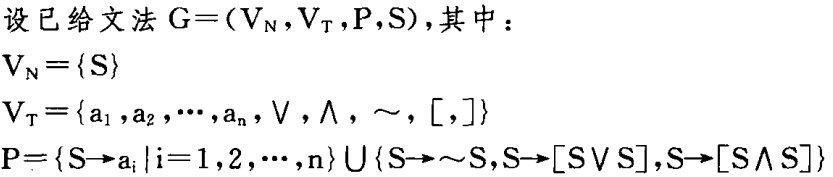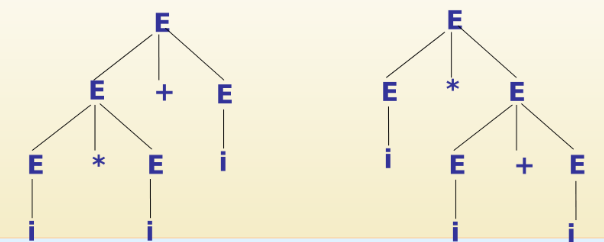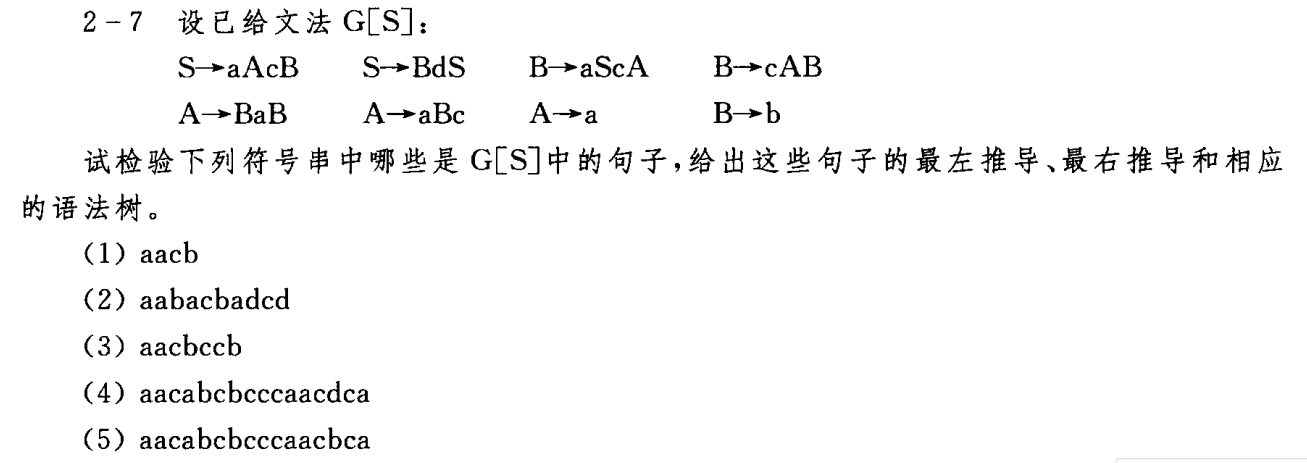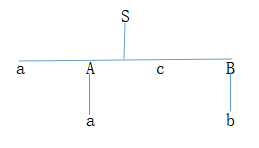## Context-free grammar and language

1. Formal representation of grammar and language

Each programming grammar has its own grammar. In fact, any program can be regarded as a character string in a certain character set , and whether a character string is a legal program in the program is determined based on It is the grammar of the language.

The grammar of a language is a set of rules, including lexical rules and grammatical rules.

Lexical rules describe the rules for structuring language word symbols . Word symbols include: identifiers, constants, operators, etc. The description tool of lexical rules is usually formal grammar ( normal form , finite automata).

The grammatical rules describe the constitutional rules of language grammatical units . The grammatical units include: expressions, statements, functions, procedures, etc. The description tool of grammar rules is often: context-free grammar .

Grammar is a formal rule describing the structure of a language. It is generally divided into four types: Type 0, Type 1, Type 2, and Type 3. Wherein the type 2 is the context-free grammar is an effective tool to describe programming language syntax , i.e., Type 3 regular grammar, it is an effective tool to describe the programming language lexical.

2. Formal definition of grammar and language

Definition 1: Production (or rule)

A production is an ordered pair (A,α), usually written as A→α (or A::=α), where A is called the left symbol of the production, and α is the finite symbol string on the right of the production , "→" means "defined as" or "consisting of".

Definition 2: Grammar

The grammar is a four-tuple G[S]=(Vn,Vt,P,S), where Vn is a non-empty finite set, each element of which is a non-terminal symbol, and Vt is a non-empty finite set, each The element is a terminal symbol. It can be seen from the definition that Vn∩Vt=Φ, the grammar symbol set V=Vn∪Vt, P is a finite set of productions, and the form of each production is: A→α, A∈Vn,α∈ V*, S is the beginning symbol S∈Vn of the grammar.

Definition 3: Derivation and reduction

Suppose G[S]=(Vn,Vt,P,S) is a given grammar, x,y,A,β∈V*, and A→β∈P, then the symbol string xAy can directly produce the symbol string xβy , We call the symbol string xβy a direct derivation of the symbol string xAy, or the symbol string xAy is a direct reduction of the symbol string xβy, denoted as xAy⇒xβy.

Definition 4: sentence patterns and sentences

Let G[S]=(Vn,Vt,P,S) be the given grammar

If S ⇒ α, α∈V , then α is called the sentence pattern of grammar G.

If S⇒+α,α∈Vt*, then α is called the sentence of grammar G.

For example: <unsigned integer>, <number string>, <number string><number>, <number><number>, 5<number>, 56 and so on are all grammatical sentence patterns . And 7, 78, 98, 1212, 32343 are all grammatical sentences.

Definition 5: Language

The set of all sentences produced by grammar G[S] is called the language defined by grammar G[S], denoted as L(G[S]), that is, L(G[S])={w|w∈Vt* , And S⇒*w}.

For example:Find the language produced by this grammar.

From the production P, we can know that the language produced by this grammar is a Boolean expression string with terminal a1, a2,...an as the operand, ∧∨ ~ as the operator, and [,] as the separator.

Definition 6: Norm Derivation and Norm Statute

For direct derivation xAy⇒xβy, if y is a Vt string or an empty symbol string , then this derivation is called a canonical derivation (the rightmost derivation); the corresponding statute is called a canonical statute (the leftmost statute)

For example: canonical derivation process
<unsigned integer>⇒<number string>
⇒<number string><number>
⇒<number string>6
⇒<number>6
⇒66 At the same time, the sentence pattern obtained by the standard derivation is called the standard sentence pattern
(Rightmost sentence type)

Two conclusions of formal language theory
(1) Given a grammar G, its language can be uniquely determined structurally, namely G→L(G)
(2) Given a language L(G), its grammar can be determined, But its grammar is not unique. That is L→G1 or G2...

Definition 7: Equivalent grammar

G1 and G2 are two different grammars. If L(G1)=L(G2), then G1 and G2 are called equivalent grammars.

Definition 8: Recursive production and recursive grammar

(1) Recursive production : For the grammar G=(Vn,Vt,P,S), if A→α∈P, and α⇒*xAy, then the production A→α is a recursive production.
( 2) Recursive grammar : A grammar containing recursive productions is called recursive grammar. The language set L(G) defined by recursive grammar is infinite, that is, recursive grammar can describe infinite languages ​​with finite productions .

Definition 9: phrase, direct phrase, and handle

Let G[S]=(Vn,Vt,P,S) be a given grammar, U∈Vn, x,y,u∈V*,

(1) If there is S⇒*xUy⇒+xuy, then u is said to be a phrase of the sentence pattern xuy relative to the non-terminal symbol U , especially if there is S⇒*xUy⇒xuy, then u is said to be the sentence pattern xuy relative to U Direct phrase . Where * means derivation above zero steps, + means derivation above one step.

(2) The leftmost direct phrase of the sentence pattern is called the handle of the sentence pattern.

For example:
<unsigned integer>⇒<number string>
⇒<number string><number>
⇒<number string>6
⇒<number>6
⇒66
6 is the sentence pattern <number>6 relative to the phrase <number >
> 6 is the sentence <number> 6 relative <digit string> phrase
<number> is the sentence <number> 6 relative <digit string> directly the phrase, and the handle is

Definition 10: Ambiguity of grammar

If there is a sentence in the grammar that corresponds to two different grammar trees, it is called an ambiguous grammar.

For example: the production set of the grammar G[E] is as follows: E→ E + E | E * E | (E) | i
The grammar tree of the sentence i * i + i has the following twocomprehensive trainingsolutions: (1) aacb Is a sentence in G[S].
The leftmost derivation is as follows:
S⇒aAcB
⇒aacB (derived from A→a)
⇒aacb ( derived from B→b)
The rightmost derivation is as follows:
S⇒aAcB
⇒aAcb (derived from B→b)
⇒aacb ( derived from B→b) A → a deduction) The
grammar tree is as follows: thedirect phrases are as follows:
a is the handle of the sentence pattern aacb relative to A;
b is the phrase
of the sentence pattern aacb relative to aAcB b is the direct phrase of the sentence pattern aAcb relative to aAcB

### Guess you like

Origin blog.csdn.net/qq_25036849/article/details/106347629
Recommended
Ranking
Daily